# Stock Valuation And The Gordon Growth Model

Feb. 25, 2016 11:11 AM ETSPY, SH, SSO4 Comments

## Summary

• The model allows investors to determine the intrinsic value of a stock based on the relationship of the dividend growth rate and the required rate of return.
• The model basically lets you strip away any current market factors and focus on the fundamentals of a company's stock.
• This article provides the in-depth understanding of the core formula and presents Excel spreadsheets so investors can obtain a valuation quickly and efficiently.

Valuing Stocks with the Gordon Growth Model

Preface

Hedge funds and financial analysts typically use a variety of approaches to determine the intrinsic value of shares. With that being said, the Gordon Growth Model is a subcategory of a larger group of mathematical models commonly known as the dividend discount models. The idea of the model states that the value of a stock is the expected future sum of all of the dividends. The model, named after Myron J. Gordon, is quite fascinating since it provides a relatively simple yet intuitive method of valuing the intrinsic value of a stock to be compared to the current market price. In this article, we will be discussing the core formula and how it serves as a benchmark for short or long decisions. Afterwards I will give insight on how to setup an Excel spreadsheet to perform the calculations so that the valuation can be obtained within a matter of seconds.

Assumptions

Before we begin the valuation, there are some assumptions that a stock must fulfill in order for this model to function properly. The assumptions are:

1. Gordon Growth Model allows you to disregard all current market factors and focus strictly on the fundamentals. With that being said, the model does not account for special products or branding that may allow a company to stand out relative to its competitors.
2. The model assumes that the stock of the company in question has and will pay dividends. Certain variations of the model work around this, however, the one presented in this article must include a stock that historically pays dividends.
3. The expected dividend growth rate must be smaller than the expected rate of return. If this is not the case, then the valuation would be negative, which is impossible (Those terms will be explained once we discuss the core formula).
4. The dividend growth rate must be estimated. Although this seems risky, I will provide information explaining what and how a dividend growth rate is estimated and how it can be done easily on Excel.
5. The dividend growth rate is assumed to be constant.
6. The sensitivity of the difference between the required rate of return and the dividend growth rate is quite high. This means one small alternation in the difference can lead to a radically different valuation (So we must be careful).

The Formula

Now that the boring rules are out of the way, it is time to take a look at the formula behind the Gordon growth model's intrinsic valuation of a stock.

First, let's denote the current value of the dividend as "D0" and a constant dividend grow rate by "g". The dividend in "n" number of years will be represented as the following: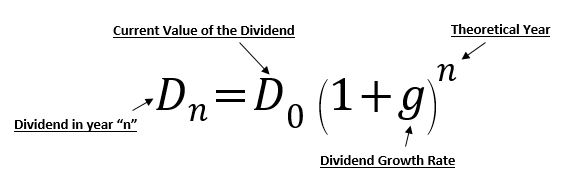Assuming now that we require some compounded rate of return represented by the variable "r", we simply take the ratio from the previously mentioned dividend in "n" year's equation shown below (See next header for calculations of "r"):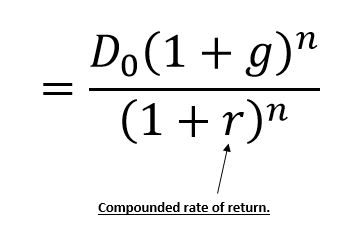If we now add every single dividend from year "n" back to the present day, we reach a total present value resembling the following: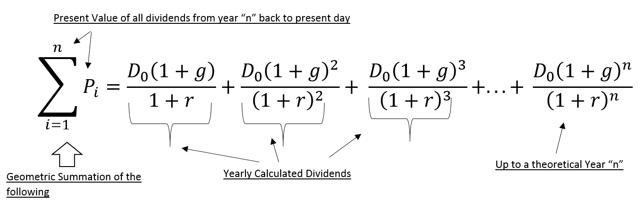If we then project this geometric series equation to "n=infinity", we can rewrite the above in the following infinite sum (We can think of this as a simpler version of the above series):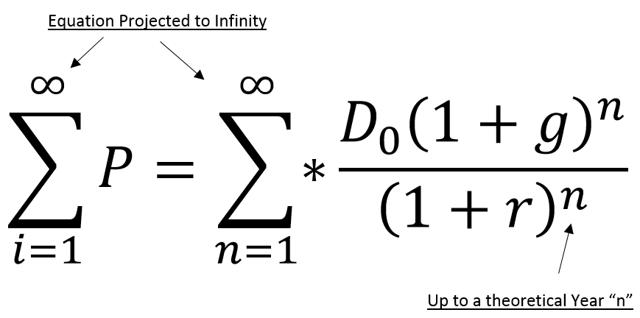Interestingly, if we extract the geometric series portion of the above equation, we are left with a remarkably simple formula, representing the intrinsic value of a stock as the following: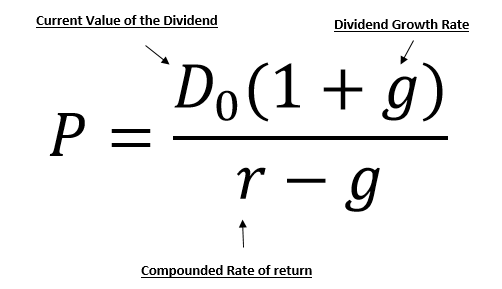If the expected dividend of (let's say) year 1 has already been determined (calculated), then the equation can be further simplified to simply the following: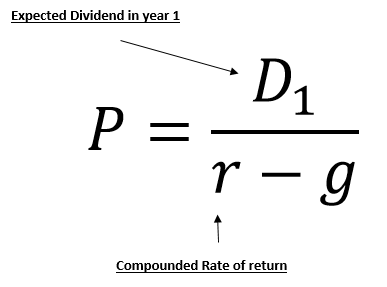Deriving the Dividend Growth Rate

Now it's time to define the way in which we obtain "g" or the dividend growth rate. First, we must acquire historical dividend data (Preferably from Yahoo). Second, we add up the quarterly dividend data per year in order to give us annualized or "yearly" data. Third, we input the relevant information into the equation below.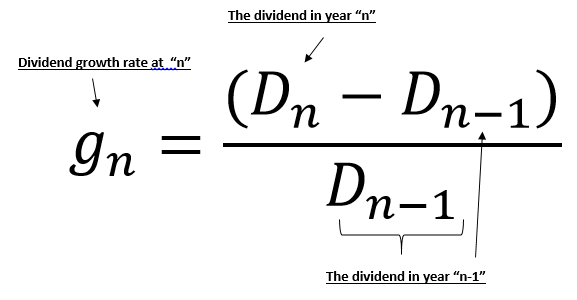Once "g" has been obtained, we then proceed to calculate the arithmetic average of the annual dividend, which can be done as follows: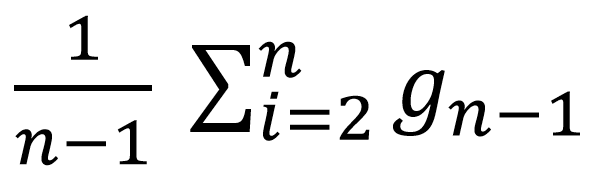This process is easily done in Excel since the summations of sets of data can easily be calculated and tweaked if needed (For information on how to calculate the dividend growth rate and even a compounded dividend growth rate, refer to the Excel guidelines in the final header).

Obtaining Required Rate of Return

Although the model is pretty intuitive, variables such as "r" representing the required rate of return must be calculated separately before being integrated in the formula. To calculate the value of "r", refer to the equation below: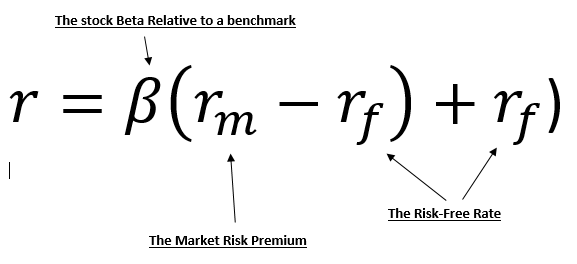The values of the variables are determined by the user. Generally, they are obtained through the preferences and research done by the user. For example, "rf" or the "risk-free rate" is generally a US Daily Treasury long-Term Rate, however, it is up to the user to make such a decision. This is therefore the same for determining the "market risk premium". The "the stock beta" on the other hand must be calculated by the user with results being relative to a certain benchmark. Ok, cool, so how does one calculate Beta?

Calculating Beta (β)

When we talk about beta being relative to a benchmark, we are specifically highlighting the geometric slope of the closing prices of a stock in a given period relative to those closing prices of popular index within the corresponding exchange. For example, shares of Exxon Mobil (XOM) can be compared to the S&P 500 as a benchmark.

When calculating beta, I personally gather 37 monthly prices (for the chosen ticker and Index) from a specific start date to an end date (You can pick as many monthly prices as you wish). In my preferred calculations, returns will be calculated from months 2 to 37 since in order to calculate the return for month 2, I need month 1 and therefore I would need 37 prices in order to get 36 results. Returns for a specific number of months, denoted as "n", can be calculated with the equation below: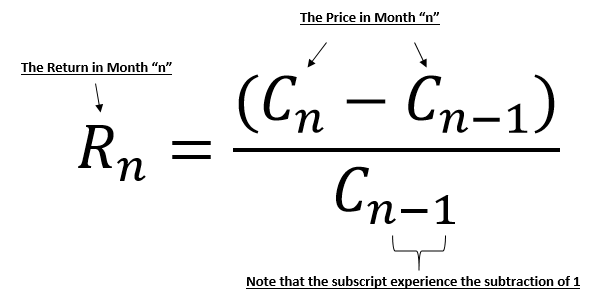To be specific, let's note that the monthly prices should be the close price on the first trading day of each month (This may not be the first day of each month). Once we have the returns for our ticker and our index, we can now calculate the Beta by using Excel's Slope function to make the calculation easier and quicker.

Calculating the Gordon Growth Model in Excel

The Excel spreadsheets that I am providing calculate the dividend growth rates, the Beta of selected stocks and the Gordon Growth Model valuation of a stock. There is one separate spreadsheet used for calculating Beta and another one which includes both the dividend growth rate and the Gordon Growth Model valuation integrated together.

Essentially, a potential investor begins by opening the "calculate beta" worksheet and inputs the respective parameters. The user must input the desire stock ticker, benchmark index ticker and both start and end dates.

The user then clicks the "Download Data button" and receives the respective information in the "results" section of the current sheet. This process is all powered by sophisticated VBA coding, allowing for the spreadsheet to download data from Yahoo Finance and make the appropriate calculation. A user should end up with the following:

Once a potential investor has the relevant Beta calculation, he or she can copy and paste that value into the next worksheet. By opening the "Gordon Growth" worksheet, the user can insert the Beta calculation in the parameters section of the Gordon Growth Model section.

Within the Gordon Growth Model spreadsheet, the investor begins by entering the respective stock in the ticker section and proceeding to click the "get bulk dividend button" in order for the VBA coding to provide both a dividend growth rate and a compounded dividend growth rate in the preceding sheets. View the graphic below:

Once the user obtains their required dividend growth rate, they can now input the given result into the Gordon Growth Model parameters. By inserting the remaining information listed to the right of the parameters section, the investor obtains a theoretical valuation in the "results" section.

This theoretical value represents the intrinsic value of the stock, and by comparing such a value with the current market price, the investor can determine whether they believe the stock is over or underpriced. That is to say, if the predicted value is higher than the actual trading price, then the share is priced fairly.

However, if the predicted value is lower than the current stock price, then the Model predicts the stock to be overpriced. Keeping those results in mind, this model can now serve as a benchmark in determining the sentiment that an investor would have towards a long or short position for a given share price.

Side Notes

This process generally takes me less than two minutes to input and calculate. I have tested these spreadsheets on my phone and they work just fine. Mobile investors will especially find the spreadsheets of this model useful for when they are on the move.

Follow

Disclosure: I/we have no positions in any stocks mentioned, and no plans to initiate any positions within the next 72 hours. I wrote this article myself, and it expresses my own opinions. I am not receiving compensation for it. I have no business relationship with any company whose stock is mentioned in this article.

Additional disclosure: I do not claim ownership of the mathematical formulas used. I am simply writing this article to provide the community with a better understanding of the model and the techniques that can be used in excel. I am not the original creator of the excel files, I simply edited them to fit with the functions of this mathematical model